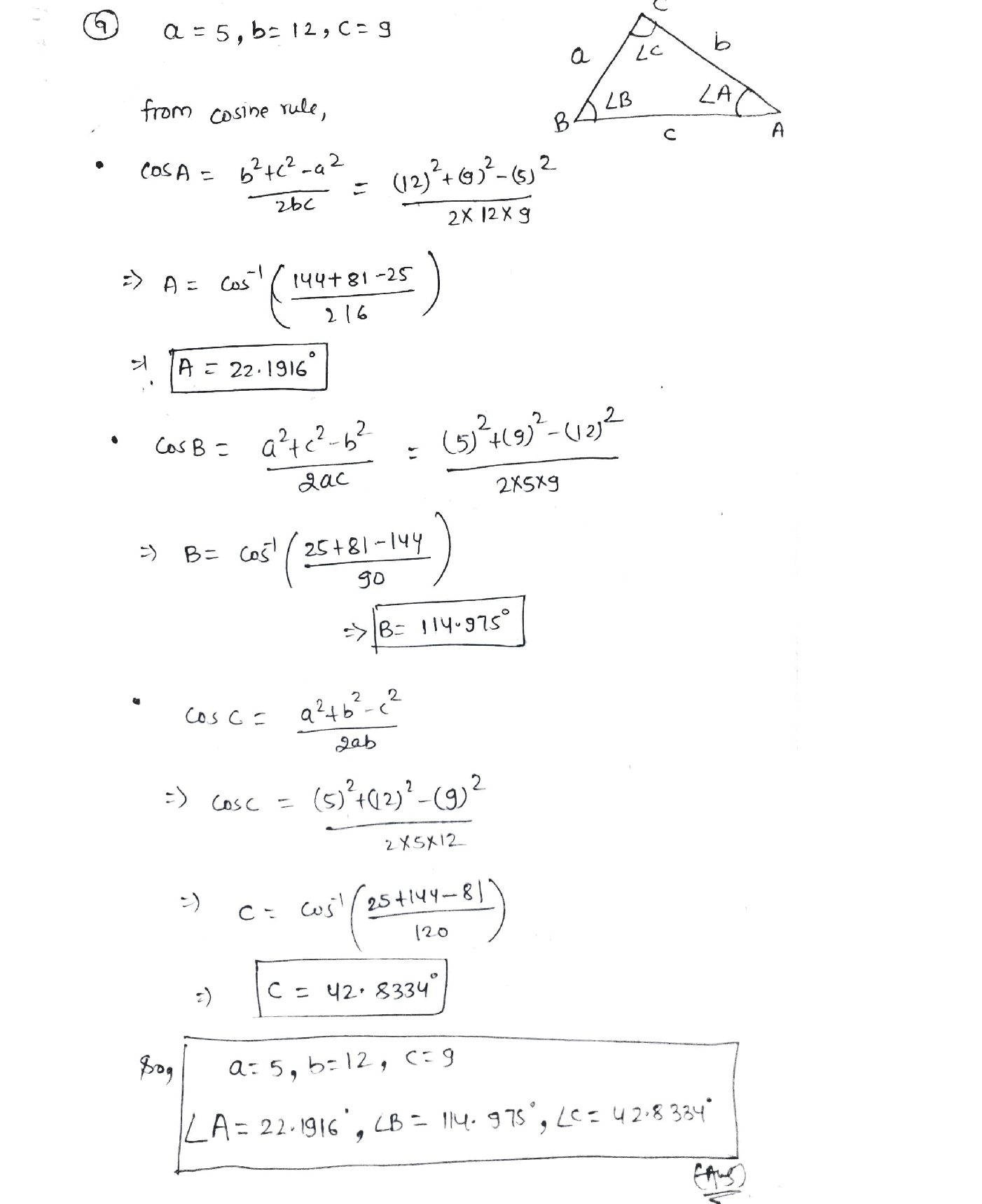Question

Trignometry

Solve the triangle with the following parts:

a=5, b=12, c=9Verified### Question 44995Trignometry

D. Sketch at least two full periods for the graph of y=2cos (4x -n)+3 on the grid below. Label the midline intercepts and the maximum and minimum points.

### Question 44993Trignometry

The range of the trigonometric function h(t) = asin(t)+d is [-16,–1]. Determine the values of a and dto the nearest tenth.

### Question 44991Trignometry

\text { Given that } \tan \theta=\frac{3}{4} \text { and that } \pi \leq \theta \leq 2 \pi
\text { a) Determine the exact value of } \sin \theta \text { and } \sec \theta \text {. }
\text { Detenmiine the value of } \theta \text {, to the nearest hundredth of a radian. }

### Question 44989Trignometry

\text { If the point }\left(x,-\frac{5}{9}\right) \text { lies on the unit circle in the third quadrant, determine the exact value of } x \text {. }

### Question 44987Trignometry

If the point (-4, 2) lies on the terminal arm of an angle 0 in standard position, determine the exact value of
\csc \theta

### Question 44986Trignometry

\text { The measure of an angle } \theta \text {, drawn in standard position below, is } \theta=\frac{6 \pi}{5} \text {. The value of the reference }
angle, is

### Question 44796Trignometry

a) Choose a point in the 3rd quadrant, that will represent a point on the terminal arm of an angle, 0 in the standard position. Determine the measure of 0 in both radians and degrees.
b) Calculate the value of 0 + 2. Determine the coordinates of the point on the new terminal arm.

### Question 44795Trignometry

a) Draw one of the primary trigonometric functions on the domain [0, 27]. Then also draw its reciprocal function.
b) List the locations of vertical asymptotes, locations of zeros, locations of minimums of your two functions drawn in 2(a).

### Question 44794Trignometry

a) Draw a sinusoidal function (not necessarily based on Sine), that has a(n):
Equation of axis greater than 1
Amplitude that is greater than 2
Period, P, where 0 < P <n
Phase shift of more than -2
O Determine 2 sinusoidal functions where
the first function has a parent function of f(x) = sin(x)
the second function has a parent function of f(x) = cos(x)

### Question 44084Trignometry

You are the architectural technician for the firm designing a new building. You need to calculate the width for a parking lot that will surround a building based on customer specifications. The building that measures90 m by 60 m is to be built on the lot and a paved parking lot of uniform width will surround the building.The paved lot is to have an area of 9000 m. Answer the questions that follow.a) How wide is the paved area? Round to the nearest tenth of a metre. (AP7)

### Submit query